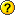## Evaluating Distributional Forecasts

Questions and discussions on Time Series Analysis

### Re: Evaluating Distributional Forecasts

If your simulations are coming in with similar results to the analytical forecasts, then the "directional accuracy" should also have similar results. If they don't, then you are probably doing the calculation wrong when inferring directional accuracy from the analytical results.
TomDoan

Posts: 7385
Joined: Wed Nov 01, 2006 5:36 pm

### Re: Evaluating Distributional Forecasts

I would note that one of the methods you are using is Normal simulations. Given a high enough number of simulations, that should converge to the "analytical" solutions. Normal simulations are useful if you are doing something that requires calculations across forecast horizons (such as, P(min value < x over range [t0,t1]), but for looking only at single forecasts will differ from analytical values only by experimental errors.
TomDoan

Posts: 7385
Joined: Wed Nov 01, 2006 5:36 pm

### Re: Evaluating Distributional Forecasts

Thanks.

TomDoan wrote:Why are you using two DO loops rather than two SET instructions? Neither one of those is saving the calculations into different slots in a time series. You can use this for the LPDS:

set scores_rwwd iend+1 iend+steps = %LOGDENSITY(stderrs_rwwd^2,y-yhat_rwwd)
sstats(mean) iend+1 iend+steps scores_rwwd>>lpds_rwwd
disp 'lpds_rwwd' lpds_rwwd

Note, BTW, that the LPDS measure is likely to favor forecasting procedures which get a better value of the variance, than a better value of the mean.

The above is for a normal distribution.

Using the transformations from @BJTRANS, what would be the LPDS for
(a) a log-normal distribution?
(b) a non-central chi-squared distribution?

LPDS is just an average of the densities of each forecast distribution. For (b) I have tried the following:

Code: Select all
`set ncsq iend+1 iend+12 = yhat_D^2.0sstats iend+1 iend+12 ncsq>>ncset scores iend+1 iend+12 = %chisqrncdensity(stderrs_D,%ndf,nc)sstats(mean) iend+1 iend+12 scores>>lpdsdisp *.############ 'lpds' lpds`

yhat_D and stderrs_D are the forecasts and forecast SE's in the square-root scale, and non-centrailty parameter nc is the sum of the squared forecasts; but get 0Please advise.
ac_1

Posts: 295
Joined: Thu Apr 15, 2010 6:30 am
Location: London, UK

Previous